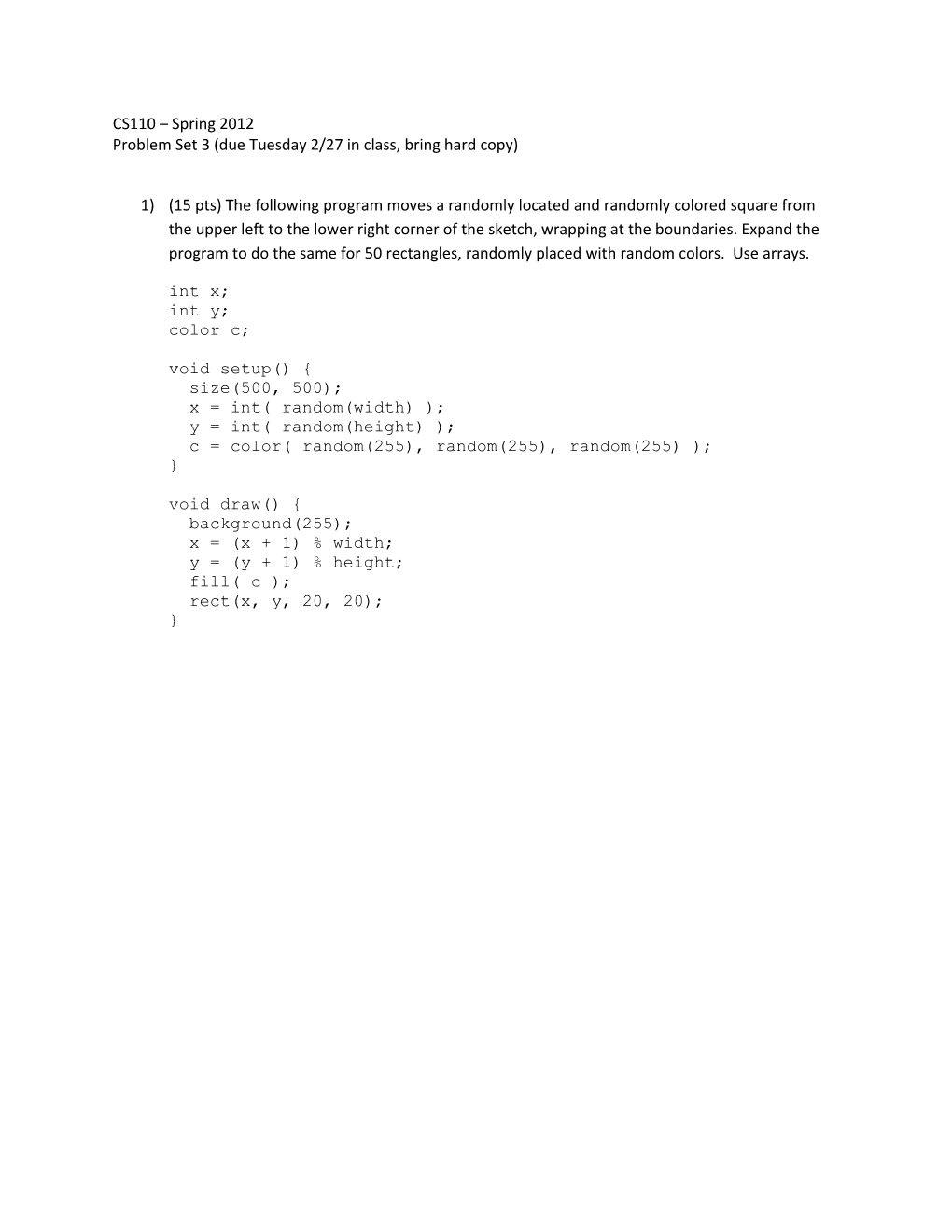Problem Set 3 (Due Tuesday 2/27 in Class, Bring Hard Copy)CS110 – Spring 2012

Problem Set 3 (due Tuesday 2/27 in class, bring hard copy)

1)(15 pts) The following program moves a randomly located and randomly colored square from the upper left to the lower right corner of the sketch, wrapping at the boundaries. Expand the program to do the same for 50 rectangles, randomly placed with random colors. Use arrays.

int x;

int y;

color c;

void setup() {

size(500, 500);

x = int(random(width));

y = int(random(height));

c = color( random(255), random(255), random(255) );

}

void draw() {

background(255);

x = (x + 1) % width;

y = (y + 1) % height;

fill( c );

rect(x, y, 20, 20);

}

2)(10 pts) Declare a float array, dimension it to hold 100 values, and fill it with random numbers. Print the numbers as they are generated and stored in the array.

3)(10 pts) Expand the previous program. After the array is fully initialized with random numbers, revisit each element in the array and multiplyeach by 2. Store each doubled value in theoriginal array location.

4)(20 pts) Modify the previous program further. Move the part of your program that doubles the values in the array to a separate function. The new function should be calleddoubleIt(). It should take a float array as its only argument and return the modified float array. The body of the function should double each element of the array. Change your program to use this new function to double the values in the original float array.

5)(45 pts) Following is a function that draws a happy face. Use this function as the basis of a new class called HappyFace. The HappyFaceclass constructor should take x, y and diamvariables that are stored in the class as fields. The class should have a method called draw() that draws itself on the sketch window. The body of the draw() method should closely follow the following happyFace() function. Test the class by creating a new instance of HappyFace positioned at the center of the sketch window and calling its draw() method.

// Draw happy face

void happyFace( float x, float y, float diam )

{

// Face

fill(255, 255, 0);

stroke(0);

strokeWeight(2);

ellipseMode(CENTER);

ellipse(x, y, diam, diam );

// Smile

float startAng = 0.1*PI;

float endAng = 0.9*PI;

float smileDiam = 0.6*diam;

arc(x, y, smileDiam, smileDiam, startAng, endAng);

// Eyes

float offset = 0.2*diam;

float eyeDiam = 0.1*diam;

fill(0);

ellipse(x-offset, y-offset, eyeDiam, eyeDiam);

ellipse(x+offset, y-offset, eyeDiam, eyeDiam);

}